# Role of Transparent Nature Conducting Substrate on Physical, Chemical and Optical Properties of Electrochemically Grown CdSe and CdSe: Fe Thin Films

Role of Transparent Nature Conducting Substrate on Physical, Chemical and Optical Properties of Electrochemically Grown CdSe and CdSe: Fe Thin Films

S. ThanikaikarasanR. Perumal R. Kanimozhi M. Saravannan P. S. Suja Ponmini

Division of Physics, Department of Science and Humanities, Saveetha School of Engineering, Saveetha Institute of Medical and Technical Sciences, Saveetha Nagar, Thandalam, Chennai 602105, Tamil Nadu, India

Department of Electronic and Communication Engineering, Annamalai University, Annamalai Nagar – 608 002, Chidambaram, Tamil Nadu, India

Department of Chemistry, Francis Xavier Engineering College, Tirunelveli – 627 003, Tamil Nadu, India

Corresponding Author Email:
s_thanikai@rediffmail.com
Page:
103-109
|
DOI:
https://doi.org/10.14447/jnmes.v25i2.a03
2 May 2021
|
Accepted:
21 March 2022
|
Published:
10 June 2022
| Citation

OPEN ACCESS

Abstract:

Semiconductors of II–VI group considered as interesting candidate for many researchers owing to its wide variety of applications in industries such as solar cells, solar selective coatings and optoelectronic devices. Chalcogenides of Cadmium received much attention due to its important structural feature, film composition, electronic and optical properties. The technique of low cost, low temperature electrochemical deposition has been employed to prepare Cadmium Selenide and Iron incorporated Cadmium Selenide thin films on transparent nature conducting substrates. The technique of X-ray diffraction has been used to identify crystalline nature and structural features of the deposited films. The method of Energy dispersive X-ray analysis has been used to find out the stoichiometric nature of the deposited films. The parameters viz., crystallite size, strain, dislocation density are estimated for the deposited films. The method of Ultraviolet-Visible spectroscopic measurements has been carried out to determine the optical properties of the deposited films. The deposited films found to exhibit band gap value in the range between 1.67 and 1.74 eV and the value of optical parameters refractive index and extinction coefficient, were estimated.

Keywords:

Band gap, CdSe: Fe, strain, dislocation density, X-ray diffraction

1. Introduction

Semiconductors are very important and interesting candidates because of their technological applications in optoelectronics, microelectronic devices like photodiodes  light emitting diodes , solar cells , photovoltaic cells and photo detectors [4, 5]. In recent times, much attention has been given to the growth of II-VI group binary semiconductors due to its wide range of applications in large scale devices such as solar cells, solar selective coatings and optoelectronic devices [6, 7]. As well, Cadmium Selenide (CdSe) is found to be an excellent and interesting candidate because of its high absorption coefficient, photosensitivity, direct intrinsic band gap of 1.73 eV and also exhibits n-type conductivity . Several research reports were available regarding the growth and characterization of CdSe thin films. However, the improved device efficiencies have been recognized only by launching intragap impurity bands.This can be obtained by the process of adjusting its physical properties owing to its localized impurity levels thus enhances the incorporation of impurity atoms into the crystal lattice . Towards this direction, prominent improvements have been achieved due to the utilization of several metal ions such as In, Sb, and Zn. Bi doped CdSe (CdSe:Bi) thin films prepared on glass substrate by thermal evaporation and its structural, electrical, optical properties are determined by Santhosh et al . Optical properties showed that there is no shift in value of band gap Bi incorporated CdSe. Vanita S Rault et al  reported that the room temperature synthesis of Indium doped CdSe (CdSe: In) thin films by electrochemical deposition and investigated the electrochemical impedance spectroscopy, spectral response measurements, and photo electrochemical properties . Growth of Sb incorporated CdSe (CdSe:Sb) thin films by electron beam evaporation and its physical and chemical properties were reported by Mathuri et al . They have reported that the deposited films have crystallite size value in the range between 40 and 50 nm. Optical properties showed that the band gap value is in the range between 1.74 and 2.3 eV and lower value of resistivity for CdSe:Sb thin films.The influence of doping on structural, optical and electrical properties of CdSe:Zn thin films prepared by thermal evaporation method have been reported by Kriti Sharma et al. . They have shown that doped films with 1 % and 5 % Zn exhibited n-type nature which has been determined by Hall Effect method. Iron doped CdSe (CdSe:Fe) thin film received considerable attention due to its potential application in solar cell and the incorporation of Fe into the lattice of CdSe thus tune the band gap of CdSe to facilitate very interesting that drags the research interest on it. However, an electrochemical synthesize of CdSe:Fe thin film provides numerous advantages such as low cost of synthesize, low temperature, high purity, free from porosity, easy to control the thickness of the grown films, morphology and composition of the deposited films can be adjusted by readily adjusting the deposition parameters as well as concentration of the electrolytic bath [13-15]. To the best of our knowledge very few reports focussed the investigation of structural, compositional, electrical, electronic, optical, photovoltaic and photo electrochemical cell properties of electrodeposited CdSe and CdSe: Fe thin films obtained from an aqueous acidic media.

In the present investigation, we have reported that the successful growth of CdSe and CdSe:Fe thin films on Tin Oxide substrate from an aqueous acidic bath in addition with complexing agent by technique of electrodeposition. Structural features showed that the crystallinity as well as the determination of structural parameters such as crystallite size, strain, dislocation density and stacking fault probability by Debye Scherrer and Williamson Smallman methods, respectively. Ultraviolet Visible spectroscopic measurements followed by 'Tauc's plot analysis has been carried out to determine band  gap value of the deposited films. The parameters refractive index extinction coefficient, real and imaginary dielectric constants of the deposited films were determined.

2. Experimental

2.1 Electrodeposition of CdSe and CdSe: Fe thin films

CdSe and CdSe:Fe thin films were deposited on Tin Oxide substrate from an aqueous electrolytic bath consists of $\mathrm{CdSO}_{4}$, $\mathrm{SeO}_{2}$ followed by the addition of $\mathrm{FeSO}_{4}$, EDTA as dopant and complexing agent, respectively. The electrolytic bath was obtained by the process of dissolving $3.8476 \mathrm{~g}$ of $3 \mathrm{CdSO}_{4} 7 \mathrm{H}_{2} \mathrm{O}$ and $0.05548 \mathrm{~g}$ of $\mathrm{SeO}_{2}$ in $250 \mathrm{cc}$ of deionized water. The doping agent $\mathrm{FeSO}_{4}$ was obtained by dissolving $0.027801 \mathrm{~g}$ of $\mathrm{FeSO}_{4}$ in $100 \mathrm{cc}$ double distilled water. The deposition process was carried out using Potentiostat/Galvanostat (SP 50, Bio Logic, France) with three electrode cell configuration consists of Tin Oxide substrate, Platinum foil, SCE as working, counter and reference electrodes, respectively. Each $20 \mathrm{cc}$ of the two solutions $\mathrm{CdSO}_{4}$ and $\mathrm{SeO}_{2}$ forms the reaction mixture for the deposition of $\mathrm{CdSe}$. Initially, the $\mathrm{pH}$ value of the electrolytic bath was $4.0 \pm 0.1$, consequently its value was adjusted to $2.5$ $\pm 0.1$ which has been carried out simply by the addition of an appropriate lesser amount of dilute. $\mathrm{H}_{2} \mathrm{SO}_{4}$ in the electrolytic bath.

Similarly, the formation of reaction mixture containing each $20 \mathrm{cc}$ of $\mathrm{CdSO}_{4}, \mathrm{SeO}_{2}$ in addition with $10 \mathrm{cc}$ of $\mathrm{FeSO}_{4}$ and EDTA as complexing agent for the deposition of CdSe: $\mathrm{Fe}$. Initially, the $\mathrm{pH}$ value of the electrolytic bath was found to be $5.5 \pm 0.1$, which may be due to the addition of EDTA as complexing agent, thereafter its value was reduced to $3.5 \pm$ $0.1$ by the addition of an appropriate lesser amount of dilute. $\mathrm{H}_{2} \mathrm{SO}_{4}$ into the electrolytic bath. The utilized Tin Oxide substrates were well cleaned in acetone within an ultrasonic bath for $15 \mathrm{~min}$ and then cleaned in running distilled water for $5 \mathrm{~min}$, finally, they were dried in air for $15 \mathrm{~min}$ before deposition. The SCE was set up into the electrolytic bath by luggin capillary arrangement whose tip was placed as close as possible to the working electrode. The deposition potential was estimated as $-750 \mathrm{mV}$ versus $\mathrm{SCE}$ for $\mathrm{CdSe}$, while the observation of slight shift in potential $-770 \mathrm{mV}$ versus SCE for CdSe: Fe which may be due to the incorporation of $\mathrm{FeSO}_{4}$ as dopant into the electrolytic bath. The bath temperature was fixed as $75^{\circ} \mathrm{C}$ and the time of deposition was found to be in the range between 5 to 60 minutes.

2.2 Characterization

Powder X-ray diffractometer (XPERTPRO PANalytical, Netherlands) with $\mathrm{CuK}_{\alpha}$ radiation $(\lambda=1.540Å)$ was used to determine the structural properties. The method of weight difference was used to estimate the thickness of the deposited films. Film composition was analyzed using an Energy dispersive X- analysis set up attached with Scanning electron microscope (JEOL JSM 840). X-ray line profile analysis was used to estimate the structural parameters such as crystallite size, rms microstrain, dislocation density, stacking fault probability, respectively. Optical absorption analysis was also carried out using an UV-Visible-NIR spectrophotometer (Shimadzu Model 200, Singapore) within the wavelength range between 300 and $1100 \mathrm{~nm}$. The parameters such as band gap, absorption coefficient, refractive index and extinction coefficient were determined.

3. Results and Discussion

3.1 Electrochemistry of CdSe and CdSe: Fe

The basic chemical reactions for the electrodeposition of CdSe and CdSe: Fe thin films and their corresponding Nernst relations are

\begin{aligned} 3 \mathrm{CdSO}_{4} 8 \mathrm{H}_{2} \mathrm{O} & \rightarrow 3 \mathrm{Cd}^{2+}+\left(\mathrm{SO}_{4}^{2-}\right)_{3}+8 \mathrm{H}_{2} \mathrm{O} \quad E_{C d}^{0}=-240 \mathrm{mV} \\ E_{C d} &=E_{C d}^{0}-\left[\frac{R T}{2 F}\right] \ln \left[\frac{a_{C d}}{a_{C d}^{2+}}\right] \end{aligned}    (1)

The formation of selenous acid occurs which may be due to the following Eq.(2)

$\mathrm{H}_{2} \mathrm{O}+\mathrm{SeO}_{2} \rightarrow \mathrm{H}_{2} \mathrm{SeO}_{3}$    (2)

Reduction of H2SeO3 to Se occurs according to the following Eq.(3)

$\mathrm{H}_{2} \mathrm{SeO}_{3}+4 \mathrm{H}^{+}+6 e^{-} \rightarrow \mathrm{Se}^{2-}+3 \mathrm{H}_{2} \mathrm{O}$

$E_{S e}=E_{S e}^{0}-\left[\frac{R T}{2 F}\right] \ln \left[\frac{a_{S e}}{a_{S e O_{2}}}\right]+\left[\frac{R T}{4 F}\right] \ln \left(a_{H}^{+}\right)^{4} \quad E_{S e}^{0}=740 \mathrm{mV}$    (3)

Reaction of formation of CdSe on Tin Oxide substrate may be due to the following Eq(4) with value of Gibbs free energy

$\Delta G^{0}=-100 \mathrm{~kJ}$ mole

$\mathrm{Cd}^{2+}+\mathrm{Se}^{2-} \rightarrow \mathrm{CdSe} \quad \Delta G^{0}=-100$ kJmole    (4)

Here $a_{\mathrm{Cd}}^{2+}, a_{\mathrm{SeO}_{2}}$ are the activities of $\mathrm{Cd}$ and $\mathrm{SeO}_{2}$ in the electrolytic bath, $a_{C d}$ and $a_{S e}$ are the activities of $\mathrm{Cd}$ and $\mathrm{Se}$ atoms in the deposited films of $\mathrm{CdSe}, E_{C d}^{0}, E_{S e}^{0}$ are the standard reduction potential of $\mathrm{Cd}$ and $\mathrm{Se} . E_{C d}, E_{S e}$ are the equilibrium electrode potential of $\mathrm{Cd}$ and $\mathrm{Se}, \mathrm{F}$ is the Faraday constant, $R$ is universal gas constant and $T$ is the absolute temperature of the electrolytic bath. The deposit is formed by the solid state reaction of plated $\mathrm{Cd}$ and Se atoms with negative free energy $\Delta G^{0}=-100 k Jmole$ rather than the precipitation of $\mathrm{H}_{2} \mathrm{Se}$ and $\mathrm{Cd}^{2+}$. The reaction of formation of $\mathrm{CdSe}: F e$ takes place according to the following Eq. (5)

$(x) C d^{2+}+S e^{2-}+(1-x) F e \rightarrow C d_{1-x} F e_{x} S e$    (5)

3.2 Weight difference method

The growth and thickness of the deposited films on conducting substrates by the technique of electrodeposition which has been be governed mainly by film thickness and surface morphology [13-16]. In electrodeposition, growth and thickness of the deposited films mainly depend upon the significant parameters viz., density of current used, solution $\mathrm{pH}$, the temperature, concentration of the electrolytic bath and the time of deposition for the prepared films $[17,18]$. Thickness of the deposited films has been determined from the bulk density, difference in weight for the film deposited before and after certain intervals of time. Thickness of CdSe and the films of CdSe:Fe deposited on Tin Oxide substrate are determined by the method of weight difference using the following Eq.(6)

$t=\frac{m}{A \rho}$    (6)

## 1.jpgFigure 1. Variation of film thickness with deposition for CdSe and CdSe:Fe thin films deposited on Tin Oxide substrate

The temperature of the electrolytic bath plays a vital role to control the rate of deposition by decreasing the viscosity of the electrolytic bath during the time of deposition. The bath temperature is optimized as 75°C for smooth coating and the same value is fixed for all experiments. Variation of film thickness with deposition time for CdSe and CdSe: Fe thin films deposited on Tin Oxide substrate is shown in Figure 1. It is noted that film thickness increases linearly with deposition time and reaches its maximum value after 40 minutes. Further increasing the deposition time, the film thickness reduces slowly, hence the deposition time was fixed 45 minutes .

3.3 Structural properties

In order to study the structural properties of the deposited thin films to investigate the crystallographic phase, the overall crystalline quality and the possible texture of those electrochemically grown thin films. Figure 2 shows the XRD pattern recorded for CdSe and CdSe:Fe films deposited on Tin Oxide substrates. The diffractogram revealed number of diffraction peaks found at $2 \theta$ value of angles $23.87,25.67$, $27.05,35.03,42.02,48.82,50.72,55.83,63.82,66.23$, corresponding to the lattice planes (100), (002), (101), (102), $(110),(200),(201),(202)$ and $(210)$, respectively. It is well known that the bulk CdSe has a highly stable hexagonal (wurtzite) from room temperature to its melting point, while cubic CdSe exists at low temperature and it is the meta stable phase . In our observed pattern the diffractogram exhibit a characteristic polycrystalline hexagonal wurtzite structure. It is noted that the peak intensity observed at $2 \theta$ value $25.81$ is found to be higher than all other intensities denoted that the crystallites are preferentially oriented along (002) plane. The process of doping transition metals as impurity did not alter the crystal structure apart from change in plane orientation of films deposited on the substrate. The| deposited CdSe and CdSe:Fe thin films have hexagonal structure with lattice constant value ( $\mathrm{a}=4.299 Å; \mathrm{c}=7.010 Å$). The observed values of " $\mathrm{d}$ " spacing were found to be $\pm 0.03$ differences from standard JCPDS ICDD value for CdSe may be due to the incorporation of impurities $\mathrm{Fe}$ into crystal lattice of CdSe . The observation of similar diffraction line of CdSe and CdSe:Fe make sure that the incorporation of impurities did not alter the crystal structure, whereas the formation of alloys. The sizes of crystallites for the deposited films have been determined using Debye Scherrer formula [16-18].

$D=\frac{0.9 \lambda}{\beta \cos \theta}$    (7)

where $\beta$ is Full Width at Half Maximum of the peak position in radian, $\lambda$ is the wavelength of $\mathrm{CuK} \alpha$ target $(\lambda=1.540 Å)$ used, $\theta$ is Bragg diffraction angle at peak position in degree.

## 2.jpgFigure 2. XRD pattern recorded for CdSe and CdSe: Fe thin films deposited on Tin Oxide substrate

Line profiles in observed XRD pattern are generally due to convolution of numerous factors viz., crystallite size, strain, dislocation density and stacking faults. An aggregate of distorted crystallite and strain could affect the variance of X-ray diffraction near the tails of the peak. A careful adjustment of the background has been carried ou byt following the method given by Williamson Smallman method 

$W_{2 \theta}=\left[\frac{\lambda \sigma}{2 \pi^{2} D \cos \theta}\right]+\left(4 \tan ^{2} \theta \prec e \succ^{2}\right)$    (8)

where $\lambda$ is the wavelength of $\mathrm{X}$-ray used, $\sigma$ the angular range over which the intensity distribution is appreciable, $D$ is the crystallite size, $\theta$ is Bragg diffraction angle, $\left\langle e^{2}\right\rangle$ is the root mean square strain. Dislocation density is defined as the number of dislocation lines per unit volume of the crystal and its value is calculated using Eq.(9) 

$\rho=\frac{3.46 \prec e^{2} \succ^{1 / 2}}{d D}$      (9)

where $n$ is the number of dislocations per unit volume of the crystal, $K$ is the constant depend on strain distribution, $\left\langle e^{2}\right\rangle^{1 / 2}$ is rms microstrain, $F$ is an interaction parameter, $b$ is the burgers vector and $D$ is the crystallite size. The value of stacking fault probability is calculated using the following Eq.(10) 

$\alpha=\left[\frac{2 \pi^{2}}{45 \sqrt{3}}\right]\left[\frac{\Delta(2 \theta)}{\tan \theta}\right]$      (10)

Figure 3 shows the value of crystallite size, rms microstrain, dislocation density and stacking fault probability with film thickness for CdSe and CdSe:Fe thin films deposited on Tin Oxide substrate. It is found that CdSe films deposited on Tin Oxide substrate has higher value of film thickness with crystallite size and lower value of dislocation density when compared with CdSe:Fe film deposited on Tin Oxide substrates.

## 3.png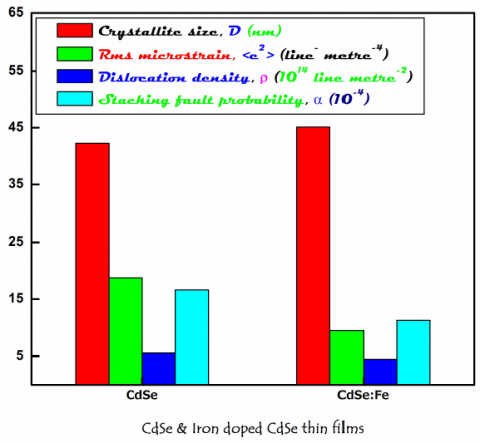Figure 3. Variation of microstructural parameters for CdSe and CdSe: Fe thin films obtained on Tin Oxide substrate

The comparison of value of Strain and Stacking fault probability with film thickness for CdSe and CdSe: Fe thin It is noted that film of CdSe has lower value of Strain and Stacking fault probability when compared with the films of CdSe: Fe deposited on Tin Oxide substrate. It has been identified that the process of doping Fe atom drastically increases the value of dislocation density and stacking faults present in the grown films. It further strengthens the enhanced crystalline quality due to the process of doping of Fe atoms into CdSe thin films that are deserved efficient for thin film devices.

3.4 Surface morphology and Film composition

The surface morphology of CdSe and CdSe:Fe thin films were analyzed using JEOL JSM 840 scanning electron microscope. SEM image of CdSe and CdSe:Fe thin films deposited on Tin Oxide substrate is shown in Figure 4a,b. The surface is found to be quite same for CdSe as well as CdSe:Fe. But, there is some few holes are present in the deposited films. The grains are observed to be smooth and covered with small spherically shaped grains. The grains are distributed uniformly over the entire surface of the substrate. The average sizes of the grains are found to be in the range between 0.8 to 1.5 μm. The addition of FeSO4 in electrolytic bath results increase in grain size of CdSe:Fe thin films as evidenced from Figure 4b.

## 4a.jpgFigure 4a. SEM image of CdSe thin film deposited on Tin Oxide substrate

## 4b.jpg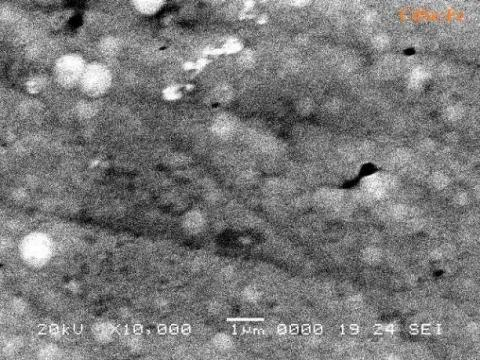Figure 4b. SEM image of CdSe:Fe thin film deposited on Tin Oxide substrate

The compositional analysis of CdSe and CdSe:Fe thin films has been carried out by EDX spectroscopy. Figure 5 shows the representative EDX spectra of CdSe and CdSe:Fe thin films deposited on Tin Oxide substrates, which also confirms the formation of alloy thin films. The elemental analysis has been carried out only for Cd, Se, Fe elements and the average atomic percentage of elements present in the deposited films.

3.5 Optical properties

Study of semiconductors by the way of optical absorption provides an information about energy band structure from the value of energy gap in eV. Optical absorbance and transmission measurements of CdSe and CdSe:Fe thin films deposited on Tin Oxide substrate was recorded within the range of wavelength in between 350 and 750 nm using Shimadzu UV-Visible-NIR spectrophotometer. Transmittance spectra recorded for CdSe and CdSe:Fe thin films deposited on Tin Oxide substrate is shown in Figure 6.

## 5.jpg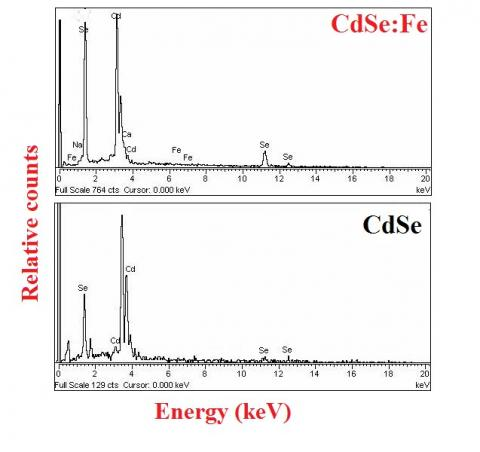Figure 5. EDX spectra of CdSe and CdSe:Fe thin films deposited on Tin Oxide substrate

## 6.jpgFigure 6. Transmittance (%) with wavelength (λ) for CdSe and CdSe: Fe thin films deposited on Tin Oxide substrate

Substrate absorption, if any is corrected by introducing an uncoated Tin Oxide substrate in the reference beam. The absorption coefficient value rises sharply owing to band-toband transition and levels off later. An analysis of absorption spectrum in the energy range between 1.0 and 3.0 eV indicates that α follows the relation

$\alpha h v=K\left(h v-E_{g}\right)^{n}$       (11)

where $(h v)$ is the photon energy in $\mathrm{eV}, E g$ is the band gap energy in $\mathrm{eV}, \alpha$ is the absorption coefficient in $\mathrm{cm}^{-1}$. For allowed direct transitions $n=1 / 2$ and for indirect transitions $n=2$. A plot of $(h v)$ versus $(\alpha h v)^{2}$ for CdSe and CdSe:Fe thin films deposited on Tin Oxide substrate is shown in Figure 9. The estimated value of band gap is found to be $1.76$ and $1.79 \mathrm{eV}$ for CdSe and CdSe:Fe. The shift in value of band gap may be due to the presence of impurity atoms as dopant in the deposited films of CdSe.

## 7.jpg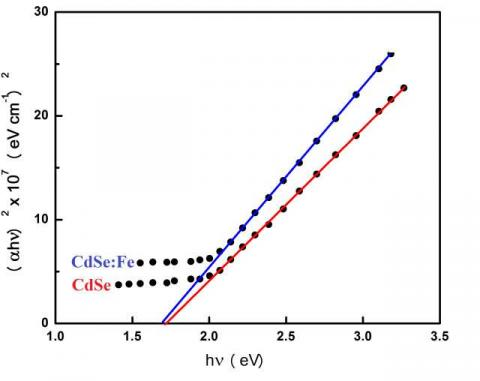Figure 7. Plot of (hν) versus (αhν)2 for CdSe and CdSe: Fe thin films deposited on Tin Oxide substrate

Refractive index is one of the basic properties of semiconductors and it is defined as the ratio of sine angle of incidence to sine angle of refraction. The value of refractive index and extinction coefficient of the deposited films has been calculated using the following Eq’s . Variation of refractive index (n) and extinction coefficient (k) with photonenergy (hν) for CdSe and CdSe: Fe for the deposited films is shown in Figure 8,9. It is observed that both of them are found to decrease if the value of wavelength increases up to 690 nm, thereafter its value remains constant.

## 8.jpg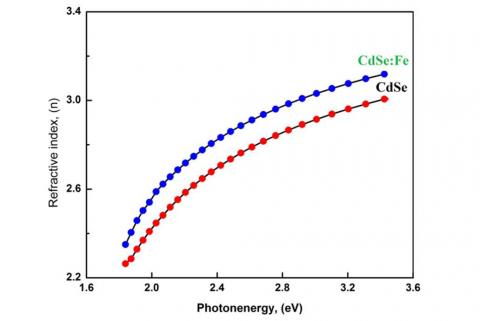Figure 8. Variation of refractive index with photon energy for CdSe and CdSe: Fe thin films

$k=\frac{\alpha \lambda}{4 \pi}$       (13)

$n=\left(\frac{1+R}{1-R}\right)+\sqrt{\frac{4 R}{(1-R)^{2}}}-k^{2}$      (14)

## 9.png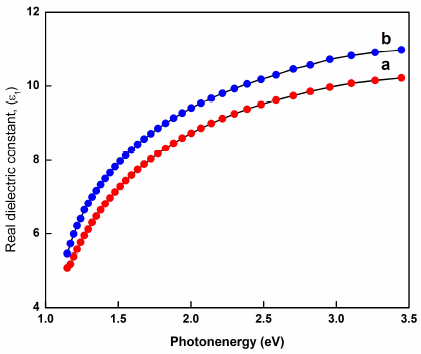Figure 9. Variation of extinction coefficient with photon energy for CdSe and CdSe: Fe thin films

The shift in value of $n, k$ for CdSe:Fe when compared with CdSe may be due to the segregation of Fe atom in electrolytic bath in the form of $\mathrm{FeSO}_{4}$ in addition with EDTA as complexing agent.

4. Conclusions

In this work polycrystalline thin films of $\mathrm{CdSe}$ and $\mathrm{CdSe}: \mathrm{Fe}$ were deposited on transparent nature conducting Tin Oxide substrate by the way of industrially applicable, low cost, low temperature electrodeposition technique. XRD results confirmed that the prepared films found to be exhibit wurtzite structure with most prominent reflection along (002) plane for the deposited film. The value of structural parameters found to exhibit monotonic variation with crystallite size whereas the remaining parameters rms micro strain, dislocation density,stacking fault probability changes vice-versa for $\mathrm{CdSe}$ and CdSe:Fe. There is slight shift in $2 \theta$ value observed which must be due to the reason that the ionic radii of $\mathrm{Fe}^{2+}$ is found to be larger than that of $\mathrm{Cd}^{2+}$. The shift in band gap value which must be due to the reason by the addition of $\mathrm{Fe}^{2+}$ as impurity atom with as-deposited CdSe. The estimated value of band gap is found to be $1.76$ and $1.79 \mathrm{eV}$ for CdSe and CdSe:Fe.

References

 Dayal S, Kopidakis N, Olson D C, Ginley D S, Rumbles G (2010) Photovoltaic Devices with a Low Band Gap Polymer and CdSe Nanostructures Exceeding 3% Efficiency.Nano Lett. 10 (1) 239-242.( DOI.10.1021/jp0734225)

 Bera S., Singh S B, Ray S K (2012). Green route synthesis of high quality CdSe quantum dots for applications in light emitting devices .J. Solid State Chem. 189, (1) 75- 79. (doi.org/10.1016/j.jssc.2011.11.048)

 Saha S K, Guchhait A, Pal A J (2012) Organic/inorganic hybrid pn-junction between copper phthalocyanine and CdSe quantum dot layers as solar cells J. Appl.Phys. 112 (4) 044507.(doi.org/10.1063/1.4747835)

 Shinde S K, Ghodake G S, Dubal D P, Lohar G M, Fulari V J (2014). Structural, optical, and photo-electrochemical properties of marygold-like CdSe0.6Te0.4 synthesized by electrochemical route J. Ceram. Int. 40 (8) 11519-11524. (doi.org/10.1016/j.ceramint.2014.03.063)

 Chatea P A, Hankareb P P, Sathe D J (2010) Characterization of cadmium selenide films for photovoltaic applications J. Alloys Compd. 505 (1) 140- 143. (doi.org/10.1016/j.jallcom.2010.06.015)

 Anandh Jesuraj S, Suganthi Devadason, Melvin David Kumar M (2017). Effect of quantum confinement in CdSe/Se multilayer thin films prepared by PVD technique. Mater. Sci. Semicond. Process. 64 (1) 109–114 (doi.org/10.1016/j.mssp.2017.03.019)

 Tanvir Hussain, Al-Kuhaili M F, Durrani S M A, Qayyum H A (2018) ). Effect of collision during vapor transport between Cd and X (X = Te2, Se2, or S2) molecules on the properties of thermally evaporated CdTe, CdSe, and CdS thin films. Results Phys. 8: 988–1000 (doi.org/10.1016/j.rinp.2018.01.042)

 Santhosh T C M , Kasturi V Bangera, Shivakumar G K (2017). Effect of Bi doping on the properties of CdSe thin films for optoelectronic device applications. Mater. Sci. Semicond. Process. 68 (1)114–117. (doi.org/10.1016/j.mssp.2017.06.004 )

 Vanita S.Raut, Chfgandrakant D Lokhande,Vilas V Killedar (2017) Photoelectrochemical studies on electrodeposited indium doped CdSe thin films using aqueous bath. J. Electroanal. Chem., 788: 137-143 (doi.org/10.1016/j.jelechem.2017.02.010).

 Mathuria S, Ramamurthi K, Ramesh Babu R (2018). Effect of Sb incorporation on the structural, optical, morphological and electrical properties of CdSe thin films deposited by electron beam evaporation technique. Thin Solid Films 660 (30) 23 - 30. (doi.org/10.1016/j.tsf.2018.05.041

 Kriti Sharma Alaa S.Al-Kabbi, Sainic G S S, Tripathi S K (2015) Influence of Zn doping on structural, optical and electrical properties of nanocrystalline CdSe thin films J Alloys Compd 651.

 Thanikaikarasan S, Dhanasekaran D, Sankaranarayanan K (2020) Electrochemical, structural, compositional and optical properties of Cuprous Selenide thin films Chinese Journal of Physics 63 (1) 138-148. /doi.org/10.1016/j.cjph.2019.10.023

 Thanikaikarasan S, Mahalingam T, Raja M, Velumani S (2015) Electrochemical growth and characterization of iron doped cadmium sulfide thin films Mat. Sci. Semicon. Process. 37, 215-222. (https://doi.org/10.1016/j.mssp.2015.03.017)

 S.Thanikaikarasan, T.Mahalingam, M.Raja, S.Velumani, (2015) Electrochemical growth and characterization of iron doped cadmium sulfide thin films, Mater.Sci. Semicon. Process. 37, 215. https://doi.org/10.1016/j.mssp.2015.03.017

 Nitu Badera, Bhavana Godbole, Srivastava S B, Vishwakarma P N, Sharath Chandra L S, Deepti Jain,Mohan Gangrade, Shripathi T, Sathe V G, Ganesan V (2008) Quenching of photoconductivity in Fe doped CdS thin films prepared by spray pyrolysis technique Appl. Surf. Sci. 254 (21) 7042-7048. doi.org/10.1016/j.mssp.2015.03.017

 Jeyakumar P, Thanikaikarasan S, B.Natarajan (2017) Role of Substrate in Electrodeposited Copper Telluride Thin Films, J Mater. Mater. Electron.28, 2538–2544 https://doi.org/10.1007/s10854-016-5828-z

 Cerdeira F, Torriani I, Motisuke P, Lemos V, Decker F (1988) Optical and structural properties of polycrystalline CdSe deposited on titanium substrates. Appl. Phys. A 46 (1) 107-112. (doi.org/10.1007/BF00615917)

 Joined Council for Powder Diffracted System, File No.08-0459, Pennsylvenia, USA.

 Mitra G B, Misra N K (1966). Background errors in X- ray diffraction parameters. Brit J Appl. Phys 17 (10) 1319-1328. (doi.org/10.1088/0508-3443/17/10/310)

 Williamson G K, Smallman R E (1956). Dislocation densities in some annealed and cold-worked metals from measurements on the X-ray debye-scherrer spectrum. Philos Mag.1:34-46. (doi.org/10.1080/14786435608238074)

 Mitra G B, Acta. Crystallogr. (1964). Determination of particle size and strain in a distorted polycrystalline aggregate by the method of variance. Acta Crystallogrica 17 (6) 765-767. (doi.org/10.1107/S0365110X6400192X)